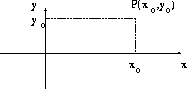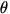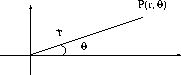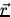# Coordinate Systems and Frames of Reference

The location of a point on a line can be described by one coordinate; a point on a plane can be described by two coordinates; a point in a three dimensional volume can be described by three coordinates. In general, the number of coordinates equals the number of dimensions. A coordinate system consists of:

1.
a fixed reference point (origin)
2.
a set of axes with specified directions and scales
3.
instructions that specify how to label a point in space relative to the origin and axes.

For Example:

• Cartesian coordinate system (rectangular coordinate system): (x,y)• Plane polar coordinates: (r,)Definition: The position vector () in any given coordinate system specifys the position of a given point within that coordinate system relative to the origin.